International
Tables for
Crystallography
Volume D
Physical properties of crystals
Edited by A. Authier

International Tables for Crystallography (2006). Vol. D, ch. 1.1, p. 11

## Section 1.1.4.2. Curie laws

A. Authiera*

aInstitut de Minéralogie et de la Physique des Milieux Condensés, Bâtiment 7, 140 rue de Lourmel, 75015 Paris, France
Correspondence e-mail: aauthier@wanadoo.fr

#### 1.1.4.2. Curie laws

| top | pdf |

The example given above shows that the symmetry of the property may possess a higher symmetry than the medium. The property is represented in that case by the indicatrix. The symmetry of an ellipsoid is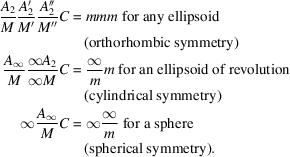[Axes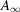are axes of revolution, or axes of isotropy, introduced by Curie (1884, 1894), cf. International Tables for Crystallography (2005), Vol. A, Table 10.1.4.2.]

The symmetry of the indicatrix is identical to that of the medium if the crystal belongs to the orthorhombic holohedry and is higher in all other cases.

This remark is the basis of the generalization of the symmetry principle by P. Curie (1859–1906). He stated that (Curie, 1894) :

 (i) the symmetry characteristic of a phenomenon is the highest compatible with the existence of the phenomenon; (ii) the phenomenon may exist in a medium that possesses that symmetry or that of a subgroup of that symmetry;

and concludes that some symmetry elements may coexist with the phenomenon but that their presence is not necessary. On the contrary, what is necessary is the absence of certain symmetry elements: asymmetry creates the phenomenon' (C'est la dissymétrie qui crée le phénomène'; Curie, 1894, p. 400). Noting that physical phenomena usually express relations between a cause and an effect (an influence and a response), P. Curie restated the two above propositions in the following way, now known as Curie laws, although they are not, properly speaking, laws:

 (i) the asymmetry of the effects must pre-exist in the causes; (ii) the effects may be more symmetric than the causes.

The application of the Curie laws enable one to determine the symmetry characteristic of a phenomenon. Let us consider the phenomenon first as an effect. If Φ is the symmetry of the phenomenon and C the symmetry of the cause that produces it,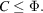Let us now consider the phenomenon as a cause producing a certain effect with symmetry E: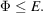We can therefore conclude that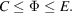If we choose among the various possible causes the most symmetric one, and among the various possible effects the one with the lowest symmetry, we can then determine the symmetry that characterizes the phenomenon.

As an example, let us determine the symmetry associated with a mechanical force. A force can be considered as the result of a traction effort, the symmetry of which is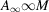. If considered as a cause, its effect may be the motion of a sphere in a given direction (for example, a spherical ball falling under its own weight). Again, the symmetry is. The symmetries associated with the force considered as a cause and as an effect being the same, we may conclude thatis its characteristic symmetry.

### References

International Tables for Crystallography (2005). Vol. A. Space-group symmetry, edited by Th. Hahn. Heidelberg: Springer.
Curie, P. (1884). Sur les questions d'ordre: répétitions. Bull. Soc. Fr. Minéral. 7, 89–110.
Curie, P. (1894). Sur la symétrie dans les phénomènes physiques, symétrie d'un champ électrique et d'un champ magnétique. J. Phys. (Paris), 3, 393–415.